# Autonomous Lawn Mower without GPS RTK

Building a few cm precision without GPS RTK.

ExpertWork in progress8,467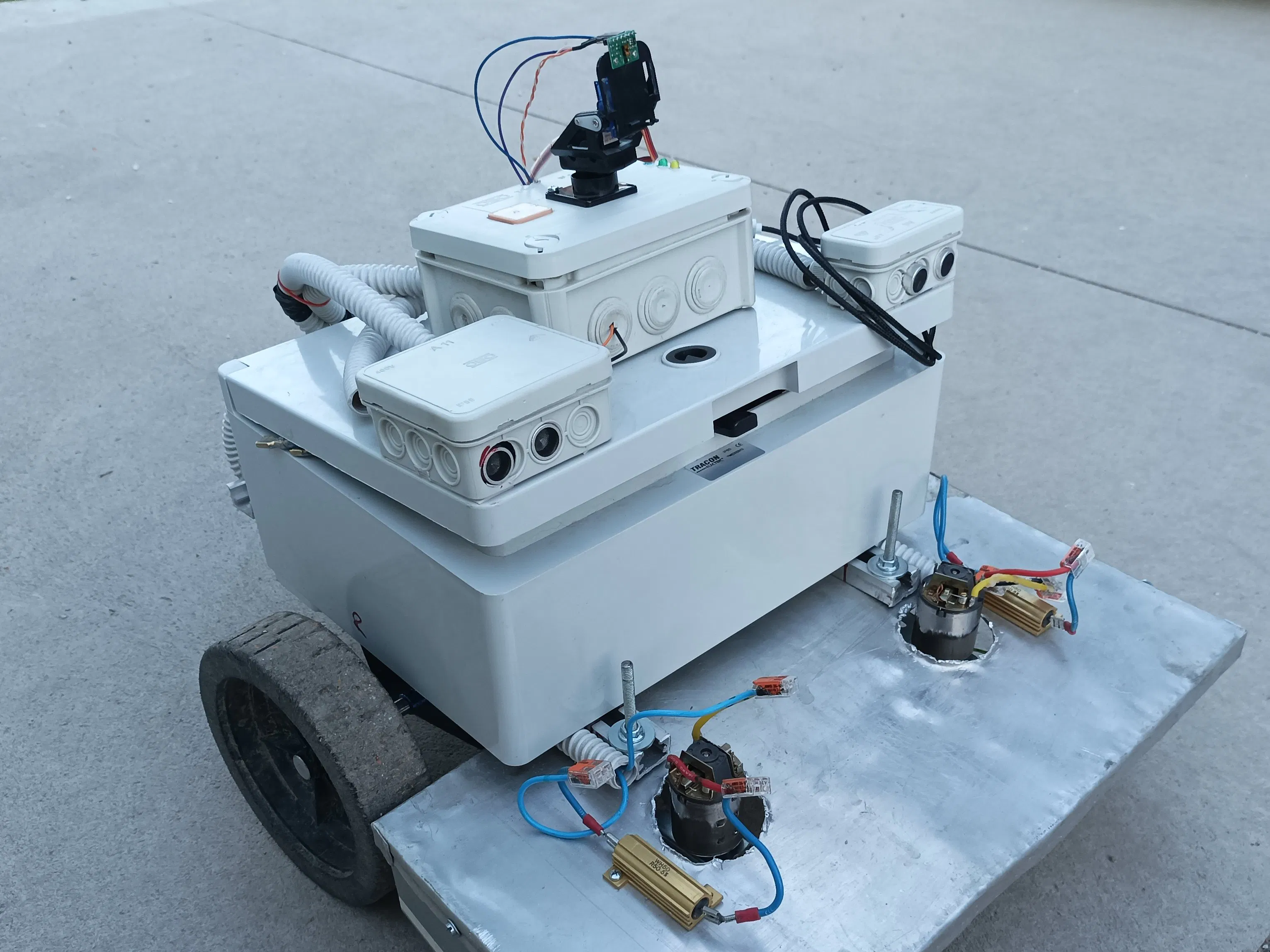## Things used in this project

### Hardware components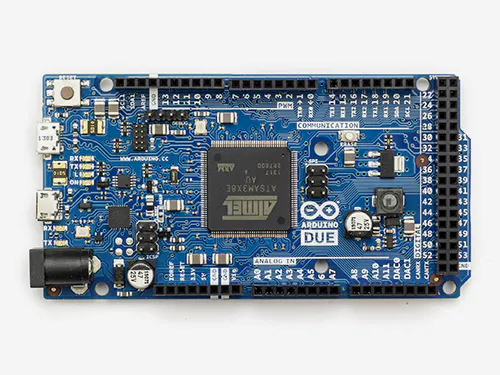Arduino Due
×1Arduino Nano R3
×2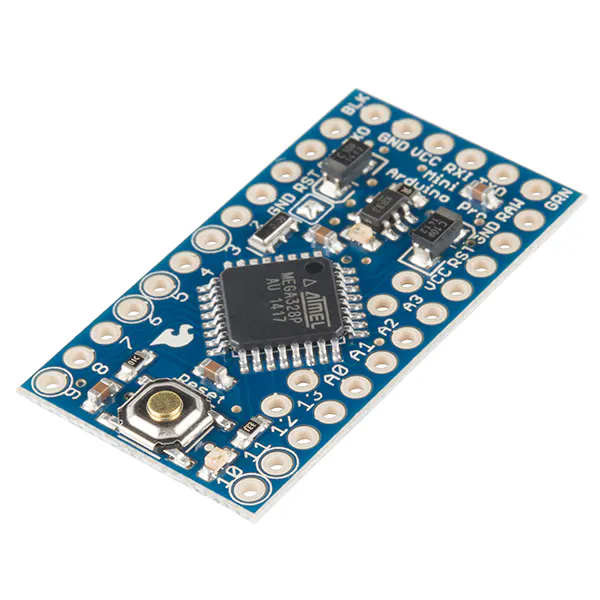SparkFun Arduino Pro Mini 328 - 3.3V/8MHz
×4Ultrasonic Sensor - HC-SR04 (Generic)
×2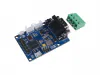Seeed Studio CANBed - Arduino CAN-BUS Development Kit (ATmega32U4 with MCP2515 and MCP2551)
×2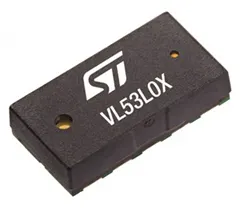STMicroelectronics VL53L0X
×1
 Qualcomm DWM1000
×1

## Code

### TAG

C/C++
It is the TAG's code. It is sending the distance values to the movement controller
```#include <SPI.h>
#include "DW1000Ranging.h"

// connection pins
const uint8_t PIN_RST = 9; // reset pin
const uint8_t PIN_IRQ = 2; // irq pin
const uint8_t PIN_SS = 7; // spi select pin

int array_lenght = 40;
bool a_active = false, b_active = false, c_active = false;
float a_distance = 1.0, b_distance = 1.0, c_distance = 1.0;
String a_string = "1.0",b_string = "1.0", c_string = "1.0", final_string = "1.0,1.0,1.0,";
unsigned int current_time = 0, last_time = 0;
float a_arr, b_arr, c_arr;
float a_avg = 1.0, b_avg = 1.0, c_avg = 1.0;

void setup() {

for(int i = 0; i < array_lenght; i++){
a_arr[i] = 1.0;
b_arr[i] = 1.0;
c_arr[i] = 1.0;
}

Serial.begin(115200);
delay(1000);
//init the configuration
DW1000Ranging.initCommunication(PIN_RST, PIN_SS, PIN_IRQ); //Reset, CS, IRQ pin
//define the sketch as anchor. It will be great to dynamically change the type of module
DW1000Ranging.attachNewRange(newRange);
DW1000Ranging.attachNewDevice(newDevice);
DW1000Ranging.attachInactiveDevice(inactiveDevice);
//Enable the filter to smooth the distance
//DW1000Ranging.useRangeFilter(true);
//Serial.println("started");

//we start the module as a tag
DW1000Ranging.startAsTag("0T:00:22:EA:82:60:3B:9C", DW1000.MODE_LONGDATA_RANGE_LOWPOWER, false);
}

void loop(){
DW1000Ranging.loop();
//Serial.println("loop");
current_time = millis();
if(current_time - last_time > 500)
{
a_avg = 0; b_avg = 0; c_avg = 0;

for(int j = 0; j < array_lenght; j++)
{
a_avg+= a_arr[j];
b_avg+= b_arr[j];
c_avg+= c_arr[j];
}

a_avg = a_avg / array_lenght;
b_avg = b_avg / array_lenght;
c_avg = c_avg / array_lenght;

if(a_active){
a_string = String(a_avg);
} else {
a_string = "Err";
}

if(b_active){
b_string = String(b_avg);
} else {
b_string = "Err";
}

if(c_active){
c_string = String(c_avg);
} else {
c_string = "Err";
}

final_string = a_string + "," + b_string + "," + c_string + "\n";
Serial.print(final_string);
last_time = current_time;
}
}

void newRange() {
float current_distance = DW1000Ranging.getDistantDevice()->getRange();
if (id == 10){update_a();   a_arr[array_lenght - 1] = current_distance; }
if (id == 11){update_b();   b_arr[array_lenght - 1] = current_distance; }
if (id == 12){update_c();   c_arr[array_lenght - 1] = current_distance; }

}

void update_a()
{
for (int i = 1; i < array_lenght; i++)
{
a_arr[i-1] = a_arr[i];
}
}

void update_b()
{
for (int i = 1; i < array_lenght; i++)
{
b_arr[i-1] = b_arr[i];
}
}

void update_c()
{
for (int i = 1; i < array_lenght; i++)
{
c_arr[i-1] = c_arr[i];
}
}

void newDevice(DW1000Device* device){
a_active = true;
b_active = true;
c_active = true;
}
}

void inactiveDevice(DW1000Device* device){
a_active = false;
b_active = false;
c_active = false;
}

}
```

### Anchor A

C/C++
Anchor A
```#include <SPI.h>
#include "DW1000Ranging.h"

// connection pins
const uint8_t PIN_RST = 9; // reset pin
const uint8_t PIN_IRQ = 2; // irq pin
const uint8_t PIN_SS = 7; // spi select pin

void setup() {
Serial.begin(115200);
delay(1000);
//init the configuration
DW1000Ranging.initCommunication(PIN_RST, PIN_SS, PIN_IRQ); //Reset, CS, IRQ pin
//define the sketch as anchor. It will be great to dynamically change the type of module
DW1000Ranging.attachNewRange(newRange);
DW1000Ranging.attachInactiveDevice(inactiveDevice);
//Enable the filter to smooth the distance
//DW1000Ranging.useRangeFilter(true);

//we start the module as an anchor
DW1000Ranging.startAsAnchor("0A:00:5B:D5:A9:9A:E2:9C", DW1000.MODE_LONGDATA_RANGE_LOWPOWER,false);
}

void loop() {
DW1000Ranging.loop();
}

void newRange() {
Serial.print("\t Range: "); Serial.print(DW1000Ranging.getDistantDevice()->getRange()); Serial.print(" m");
Serial.print("\t RX power: "); Serial.print(DW1000Ranging.getDistantDevice()->getRXPower()); Serial.println(" dBm");
}

Serial.print(" short:");
}

void inactiveDevice(DW1000Device* device) {
Serial.print("delete inactive device: ");
}
```

### Anchor B

C/C++
Anchor B
```#include <SPI.h>
#include "DW1000Ranging.h"

// connection pins
const uint8_t PIN_RST = 9; // reset pin
const uint8_t PIN_IRQ = 2; // irq pin
const uint8_t PIN_SS = 7; // spi select pin

void setup() {
Serial.begin(115200);
delay(1000);
//init the configuration
DW1000Ranging.initCommunication(PIN_RST, PIN_SS, PIN_IRQ); //Reset, CS, IRQ pin
//define the sketch as anchor. It will be great to dynamically change the type of module
DW1000Ranging.attachNewRange(newRange);
DW1000Ranging.attachInactiveDevice(inactiveDevice);
//Enable the filter to smooth the distance
//DW1000Ranging.useRangeFilter(true);

//we start the module as an anchor
DW1000Ranging.startAsAnchor("0B:00:5B:D5:A9:9A:E2:9C", DW1000.MODE_LONGDATA_RANGE_LOWPOWER,false);
}

void loop() {
DW1000Ranging.loop();
}

void newRange() {
Serial.print("\t Range: "); Serial.print(DW1000Ranging.getDistantDevice()->getRange()); Serial.print(" m");
Serial.print("\t RX power: "); Serial.print(DW1000Ranging.getDistantDevice()->getRXPower()); Serial.println(" dBm");
}

Serial.print(" short:");
}

void inactiveDevice(DW1000Device* device) {
Serial.print("delete inactive device: ");
}
```

### Anchor C

C/C++
Anchor C
```#include <SPI.h>
#include "DW1000Ranging.h"

// connection pins
const uint8_t PIN_RST = 9; // reset pin
const uint8_t PIN_IRQ = 2; // irq pin
const uint8_t PIN_SS = 7; // spi select pin

void setup() {
Serial.begin(115200);
delay(1000);
//init the configuration
DW1000Ranging.initCommunication(PIN_RST, PIN_SS, PIN_IRQ); //Reset, CS, IRQ pin
//define the sketch as anchor. It will be great to dynamically change the type of module
DW1000Ranging.attachNewRange(newRange);
DW1000Ranging.attachInactiveDevice(inactiveDevice);
//Enable the filter to smooth the distance
//DW1000Ranging.useRangeFilter(true);

//we start the module as an anchor
DW1000Ranging.startAsAnchor("0C:00:5B:D5:A9:9A:E2:9C", DW1000.MODE_LONGDATA_RANGE_LOWPOWER,false);
}

void loop() {
DW1000Ranging.loop();
}

void newRange() {
Serial.print("\t Range: "); Serial.print(DW1000Ranging.getDistantDevice()->getRange()); Serial.print(" m");
Serial.print("\t RX power: "); Serial.print(DW1000Ranging.getDistantDevice()->getRXPower()); Serial.println(" dBm");
}

Serial.print(" short:");
}

void inactiveDevice(DW1000Device* device) {
Serial.print("delete inactive device: ");
}
```

### Usonic

C/C++
Reading the Ultrasonic sensor's and sending the value over CAN to the movement controller.
```// CAN
#include<mcp2515.h>

// Usonic
#include <Usonic.h>

struct can_frame Usonic_data;
MCP2515 mcp2515(10);								// the pin for the CAN shall be defined

int distance_1 = 0, distance_2 = 0;

void setup() {

Timer1.initialize(100000);         // initialize timer1 in microseconds
Timer1.attachInterrupt(timer_interrupt);  // attaches callback() as a timer overflow interrupt

Usonic_setup();
Serial.begin(57600);

// initializing the CAN message
Usonic_data.can_id  = 0x041;    // CAN ID
Usonic_data.can_dlc = 8;      // CAN DLC
Usonic_data.data = 0x00;   // Speed value byte 0
Usonic_data.data = 0x00;   // Speed value byte 1
Usonic_data.data = 0x00;   // Speed value byte 2
Usonic_data.data = 0x00;   // Speed value byte 3
Usonic_data.data = 0x00;   // Empty byte
Usonic_data.data = 0x00;   // Empty byte
Usonic_data.data = 0x00;   // Empty byte
Usonic_data.data = 0x00;   // Life singal.

// starting the CAN
mcp2515.reset();
mcp2515.setBitrate(CAN_1000KBPS);
mcp2515.setNormalMode();

}

void loop() {
Usonic_loop();

distance_1 = Usonic_1_distance();
distance_2 = Usonic_2_distance();

Serial.print("Usonic 1: ");
Serial.print(distance_1);
Serial.print(", Usonic 2: ");
Serial.print(distance_2);

}

void timer_interrupt(){
// sending the CAN message

Usonic_data.data = ((uint8_t*)&distance_1);    // Speed value byte 0
Usonic_data.data = ((uint8_t*)&distance_1);    // Speed value byte 1
Usonic_data.data = ((uint8_t*)&distance_2);    // Speed value byte 2
Usonic_data.data = ((uint8_t*)&distance_2);    // Speed value byte 3

mcp2515.sendMessage(&Usonic_data);
}
```

### LIDAR

C/C++
Sending the actual angle of the servo and distance to the movement controller over CAN, only if the movement controller sent a Scanning signal.
```#include <TimerOne.h>
#include<mcp2515.h>
#include <Servo.h>
#include <Wire.h>
#include <VL53L1X.h>

Servo scanning;
Servo vertical;

VL53L1X sensor;

MCP2515 mcp2515(10);                // the pin for the CAN shall be defined

float LIDAR_distance = 0.0, LIDAR_angle = 0.0;
byte Life = 0, Scanning = 0, Output_1 = 0, Output_2 = 0, Output_3 = 0;
int act_scan_angle = 0, scanning_step_angle = 10, Scanning_min = 30, Scanning_max = 150, Vertical_Scan_pos = 90, Vertical_banked_pos = 150, act_distance = 0, detection_limit = 430;

void setup(){
scanning.attach(40);
vertical.attach(41);

Wire.begin();
Wire.setClock(400000); // use 400 kHz I2C

sensor.setTimeout(500);
sensor.init();
delay(200);
// Use long distance mode and allow up to 50000 us (50 ms) for a measurement.
// You can change these settings to adjust the performance of the sensor, but
// the minimum timing budget is 20 ms for short distance mode and 33 ms for
// medium and long distance modes. See the VL53L1X datasheet for more
// information on range and timing limits.
sensor.setDistanceMode(VL53L1X::Long);
sensor.setMeasurementTimingBudget(50000);

// Start continuous readings at a rate of one measurement every 50 ms (the
// inter-measurement period). This period should be at least as long as the
// timing budget.
sensor.startContinuous(50);

Timer1.initialize(100000);         // initialize timer1 in microseconds
Timer1.attachInterrupt(timer_interrupt);  // attaches callback() as a timer overflow interrupt

Serial.begin(115200);

// initializing the CAN message
Received_data.can_id  = 0x051;    // CAN ID
Received_data.can_dlc = 8;      // CAN DLC
Received_data.data = 0x00;   // Speed value byte 0
Received_data.data = 0x00;   // Speed value byte 1
Received_data.data = 0x00;   // Speed value byte 2
Received_data.data = 0x00;   // Speed value byte 3
Received_data.data = 0x00;   // Empty byte
Received_data.data = 0x00;   // Empty byte
Received_data.data = 0x00;   // Empty byte
Received_data.data = 0x00;   // Life singal.

Sent_data.can_id  = 0x061;    // CAN ID
Sent_data.can_dlc = 8;      // CAN DLC
Sent_data.data = 0x00;   // Speed value byte 0
Sent_data.data = 0x00;   // Speed value byte 1
Sent_data.data = 0x00;   // Speed value byte 2
Sent_data.data = 0x00;   // Speed value byte 3
Sent_data.data = 0x00;   // Empty byte
Sent_data.data = 0x00;   // Empty byte
Sent_data.data = 0x00;   // Empty byte
Sent_data.data = 0x00;   // Life singal.

// starting the CAN
mcp2515.reset();
mcp2515.setBitrate(CAN_1000KBPS);
mcp2515.setNormalMode();

}

void loop(){

}

Serial.print(" ");
Serial.print(" ");

for (int i = 0; i<Received_data.can_dlc; i++)  {  // print the data
Serial.print(" ");
}

Serial.println();
}

if(Output_1){
digitalWrite(2, HIGH);
} else {
digitalWrite(2, LOW);
}

if(Output_2){
digitalWrite(3, HIGH);
} else {
digitalWrite(3, LOW);
}

if(Output_3){
digitalWrite(4, HIGH);
} else {
digitalWrite(4, LOW);
}
}

void timer_interrupt(void){
if(Scanning){

if(act_scan_angle >= Scanning_max){
act_scan_angle = Scanning_min;
}

scanning.write(act_scan_angle);
act_scan_angle+=scanning_step_angle;
vertical.write(Vertical_Scan_pos);

LIDAR_angle = act_scan_angle;

} else {
scanning.write((Scanning_min + Scanning_max)/2);
vertical.write(Vertical_Scan_pos);
act_scan_angle = Scanning_min;
}

Sent_data.data = ((uint8_t*)&LIDAR_distance);    // Speed value byte 0
Sent_data.data = ((uint8_t*)&LIDAR_distance);    // Speed value byte 1
Sent_data.data = ((uint8_t*)&LIDAR_angle);       // Speed value byte 2
Sent_data.data = ((uint8_t*)&LIDAR_angle);       // Speed value byte 3
Sent_data.data = Life++;

mcp2515.sendMessage(&Sent_data);
Serial.println("CAN msg sent");
}
```

### Movement controller

C/C++
Movement controller of the mower.
```/* This could not be uploaded :-( */
```

## Credits

### Viktor Kurusa

0 projects • 4 followers